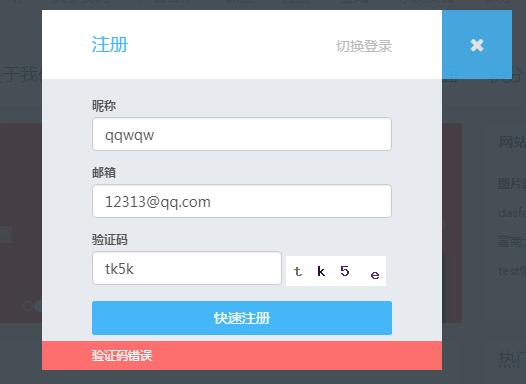# DUX主题注册新用户时增加验证码防止机器注册（适用其他主题）```<?php
/**
* 字母+数字的验证码生成
*/
// 开启session
session_start();
//创建画布
\$image = imagecreatetruecolor(100, 30);
//为画布定义(背景)颜色
\$bgcolor = imagecolorallocate(\$image, 255, 255, 255);
//填充颜色
imagefill(\$image, 0, 0, \$bgcolor);
//设置验证码内容
//定义验证码的内容
\$content = "ABCDEFGHJKLMNPQRSTUVWXYZabcdefghijkmnpqrstuvwxyz23456789";
//创建一个变量存储产生的验证码数据，便于用户提交核对
for (\$i = 0; \$i < 4; \$i++) {
// 字体大小
\$fontsize = 10;
// 字体颜色
\$fontcolor = imagecolorallocate(\$image, mt_rand(0, 120), mt_rand(0, 120), mt_rand(0, 120));
// 设置字体内容
\$fontcontent = substr(\$content, mt_rand(0, strlen(\$content)), 1);
// 显示的坐标
\$x = (\$i * 100 / 4) + mt_rand(5, 10);
\$y = mt_rand(5, 10);
// 填充内容到画布中
imagestring(\$image, \$fontsize, \$x, \$y, \$fontcontent, \$fontcolor);
}

//向浏览器输出图片头信息
//输出图片到浏览器
imagepng(\$image);
//销毁图片
imagedestroy(\$image);
?>```

dux的注册表单在主题目录下的js文件的signpop.js文件中，我们为注册表单新增一项验证码，新增HTML如下：

```<h6>\
<label for="inputEmail2"">验证码</label>\
<div>\
<img src="1.php"  onclick="this.src=\'1.php?\'+new Date().getTime();" width="100" height="30">\
</div>\
</h6>\
```

```session_start();
}else{
print_r(json_encode(array('error'=>1, 'msg'=>'验证码错误')));
exit();
}```

```//设置背景干扰元素
for (\$\$i = 0; \$i < 200; \$i++) {
\$pointcolor = imagecolorallocate(\$image, mt_rand(50, 200), mt_rand(50, 200), mt_rand(50, 200));
imagesetpixel(\$image, mt_rand(1, 99), mt_rand(1, 29), \$pointcolor);
}

//设置干扰线
for (\$i = 0; \$i < 3; \$i++) {
\$linecolor = imagecolorallocate(\$image, mt_rand(50, 200), mt_rand(50, 200), mt_rand(50, 200));
imageline(\$image, mt_rand(1, 99), mt_rand(1, 29), mt_rand(1, 99), mt_rand(1, 29), \$linecolor);
}```